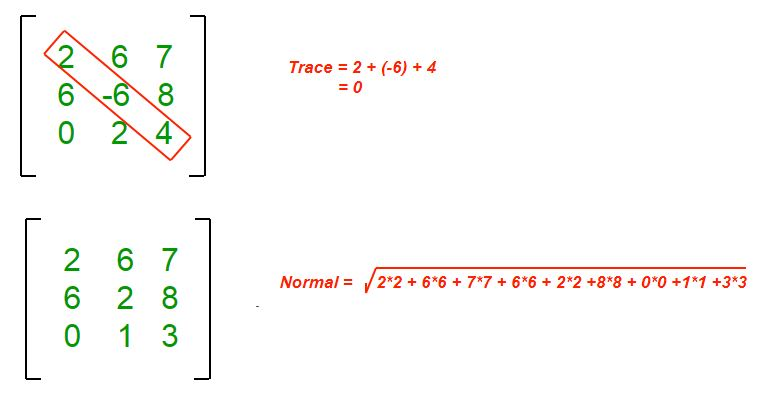# Program to find Normal and Trace of a matrix

Given a 2D matrix, the task is to find Trace and Normal of matrix.

Normal of a matrix is defined as square root of sum of squares of matrix elements.

Trace of a n x n square matrix is sum of diagonal elements.

Examples :

```Input : mat[][] = {{7, 8, 9},
{6, 1, 2},
{5, 4, 3}};
Output : Normal = 16
Trace  = 11
Explanation :
Normal = sqrt(7*7+ 8*8 + 9*9 + 6*6 +
1*1 + 2*2 + 5*5 + 4*4 + 3*3)
= 16
Trace  = 7+1+3 = 11

Input :mat[][] = {{1, 2, 3},
{6, 4, 5},
{2, 1, 3}};
Output : Normal = 10
Trace = 8
Explanation :
Normal = sqrt(1*1 +2*2 + 3*3 + 6*6 + 4*4 +
5*5 + 2*2 + 1*1 + 3*3)
Trace = 8(1+4+3)
```

## Recommended: Please try your approach on {IDE} first, before moving on to the solution.## C++

 `// C++ program to find trace and normal ` `// of given matrix ` `#include ` `using` `namespace` `std; ` ` `  `// Size of given matrix ` `const` `int` `MAX = 100; ` ` `  `// Returns Normal of a matrix of size n x n ` `int` `findNormal(``int` `mat[][MAX], ``int` `n) ` `{ ` `    ``int` `sum = 0; ` `    ``for` `(``int` `i=0; i

## Java

 `// Java program to find trace and normal ` `// of given matrix ` ` `  `import` `java.io.*; ` ` `  `class` `GFG { ` ` `  `// Size of given matrix ` `static`  `int` `MAX = ``100``; ` ` `  `// Returns Normal of a matrix of size n x n ` ` ``static` `int` `findNormal(``int` `mat[][], ``int` `n) ` `{ ` `    ``int` `sum = ``0``; ` `    ``for` `(``int` `i=``0``; i

## Python3

 `# Python3 program to find trace and  ` `# normal of given matrix  ` `import` `math ` ` `  `# Size of given matrix  ` `MAX` `=` `100``;  ` ` `  `# Returns Normal of a matrix  ` `# of size n x n  ` `def` `findNormal(mat, n):  ` ` `  `    ``sum` `=` `0``;  ` `    ``for` `i ``in` `range``(n):  ` `        ``for` `j ``in` `range``(n):  ` `            ``sum` `+``=` `mat[i][j] ``*` `mat[i][j];  ` `    ``return` `math.floor(math.sqrt(``sum``));  ` ` `  `# Returns trace of a matrix of  ` `# size n x n  ` `def` `findTrace(mat, n):  ` ` `  `    ``sum` `=` `0``;  ` `    ``for` `i ``in` `range``(n):  ` `        ``sum` `+``=` `mat[i][i];  ` `    ``return` `sum``;  ` ` `  `# Driver Code  ` `mat ``=` `[[``1``, ``1``, ``1``, ``1``, ``1``],  ` `       ``[``2``, ``2``, ``2``, ``2``, ``2``],  ` `       ``[``3``, ``3``, ``3``, ``3``, ``3``],  ` `       ``[``4``, ``4``, ``4``, ``4``, ``4``],  ` `       ``[``5``, ``5``, ``5``, ``5``, ``5``]];  ` ` `  `print``(``"Trace of Matrix ="``, findTrace(mat, ``5``));  ` ` `  `print``(``"Normal of Matrix ="``, findNormal(mat, ``5``));  ` ` `  `# This code is contributed by mits `

## C#

 `// C# program to find trace and normal ` `// of given matrix ` `using` `System; ` ` `  `class` `GFG { ` `     `  `    ``// Returns Normal of a matrix of ` `    ``// size n x n ` `    ``static` `int` `findNormal(``int` `[,]mat, ``int` `n) ` `    ``{ ` `        ``int` `sum = 0; ` `         `  `        ``for` `(``int` `i = 0; i < n; i++) ` `            ``for` `(``int` `j = 0; j < n; j++) ` `                ``sum += mat[i,j] * mat[i,j]; ` `                 `  `        ``return` `(``int``)Math.Sqrt(sum); ` `    ``} ` `     `  `    ``// Returns trace of a matrix of size  ` `    ``// n x n ` `    ``static` `int` `findTrace(``int` `[,]mat, ``int` `n) ` `    ``{ ` `        ``int` `sum = 0; ` `         `  `        ``for` `(``int` `i = 0; i < n; i++) ` `            ``sum += mat[i,i]; ` `             `  `        ``return` `sum; ` `    ``} ` `     `  `    ``// Driven source ` `    ``public` `static` `void` `Main ()  ` `    ``{ ` `        ``int` `[,]mat = { {1, 1, 1, 1, 1}, ` `                       ``{2, 2, 2, 2, 2}, ` `                       ``{3, 3, 3, 3, 3}, ` `                       ``{4, 4, 4, 4, 4}, ` `                       ``{5, 5, 5, 5, 5}, ` `    ``}; ` ` `  `    ``Console.Write (``"Trace of Matrix = "` `            ``+ findTrace(mat, 5) + ``"\n"``); ` `    ``Console.Write(``"Normal of Matrix = "` `                    ``+ findNormal(mat, 5)); ` `         `  `    ``} ` `}  ` ` `  `// This code is contributed by nitin mittal. `

## PHP

 ` `

Output :

```Trace of matrix = 15
Normal of matrix = 16
```

Time Complexity :
O(n*n)
Space Complexity : O(1)

This article is contributed by DANISH_RAZA. If you like GeeksforGeeks and would like to contribute, you can also write an article using contribute.geeksforgeeks.org or mail your article to contribute@geeksforgeeks.org. See your article appearing on the GeeksforGeeks main page and help other Geeks.

Don’t stop now and take your learning to the next level. Learn all the important concepts of Data Structures and Algorithms with the help of the most trusted course: DSA Self Paced. Become industry ready at a student-friendly price.

My Personal Notes arrow_drop_up

Article Tags :
Practice Tags :

1

Please write to us at contribute@geeksforgeeks.org to report any issue with the above content.